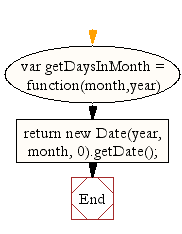# JavaScript: Get the number of days in a month

## JavaScript Datetime: Exercise-3 with Solution

Write a JavaScript function to get the number of days in a month.

Test Data:
console.log(getDaysInMonth(1, 2012));
console.log(getDaysInMonth(2, 2012));
console.log(getDaysInMonth(9, 2012));
console.log(getDaysInMonth(12, 2012));
Output :
31
29
30
31

Sample Solution:-

HTML Code:

``````<!DOCTYPE html>
<html>
<head>
<meta charset="utf-8">
<title>Get days in Month</title>
</head>
<body>
</body>
</html>
```
```

JavaScript Code:

``````var getDaysInMonth = function(month,year) {
// Here January is 1 based
//Day 0 is the last day in the previous month
return new Date(year, month, 0).getDate();
// Here January is 0 based
// return new Date(year, month+1, 0).getDate();
};
console.log(getDaysInMonth(1, 2012));
console.log(getDaysInMonth(2, 2012));
console.log(getDaysInMonth(9, 2012));
console.log(getDaysInMonth(12, 2012));
```
```

Sample Output:

```31
29
30
31
```

Flowchart:Live Demo:

See the Pen JavaScript - Get the number of days in a month-date-ex- 3 by w3resource (@w3resource) on CodePen.

Improve this sample solution and post your code through Disqus

What is the difficulty level of this exercise?

﻿

## JavaScript: Tips of the Day

Convert tabs to spaces, where each tab corresponds to count spaces

Example:

```const tips_expandTabs = (str, count) => str.replace(/\t/g, ' '.repeat(count));
console.log(tips_expandTabs('\t\tw3resource', 4));
```

Output:

```"        w3resource"
```